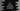# C++ program to convert octal value to decimal## C++ program to convert octal value to decimal:

In this post, we will learn how to convert an octal to decimal value. Decimal values. Decimal numbers are represented in base 10 and octal numbers are represented in base 8.

Converting a octal number to decimal means converting a base 8 number to a base 10 number.

In this post, we will learn how to convert a octal value to decimal.

### How to convert octal to decimal:

To convert an octal number to decimal, we need to follow the below steps:

• We will start from the rightmost digit to the leftmost digit.
• For each digit, we will multiply it with 8 with the power of current position, it starts from 0. For the rightmost digit, we will multiply it with 8^0, for the second digit, multiply it with 8^1 etc.
• Finally, add all these values and it will be the required decimal number.

For example, if we want to change octal 145 to decimal, we can do it as like below:

• 1 * (8^2) + 4 * (8^1) + 5 * (8^0) = 64 + 32 + 5 = 101

So, decimal representation of 145 is 101.

### C++ program:

Below is the complete C++ program:

``````#include <iostream>
using namespace std;

int octalToDecimal(int octal)
{
int decimal = 0;
int multiplier = 1;

while (octal > 0)
{
int rightDigit = octal % 10;
octal = octal / 10;
decimal += rightDigit * multiplier;
multiplier *= 8;
}

return decimal;
}

int main()
{
int num;
cout << "Enter the value in octal :" << endl;
cin >> num;

cout << "Decimal value : " << octalToDecimal(num) << endl;
}``````

Here,

• We are asking the user to enter the octal value and storing it in the variable num.
• octalToDecimal is used to convert one octal value to decimal.
• It takes the right most digit from the number and multiply with the required 8 power. The while loop in the program does that.
• It returns the decimal representation of the octal value, i.e. decimal once ends.

### Output:

This program will give output as like below:

``````Enter the value in octal :
145
Decimal value : 101``````